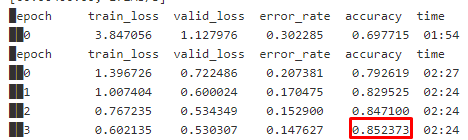# Getting Metrics on Validation Set

Hi

I am just getting started with FastAI. At the moment I am working on a small side project where I am planning to use Azure ML to deploy a CV model that can classify various fruits and vegetables from images. I created a model and trained it and all worked perfectly. I am now wondering how I can get certain metrics as plain numbers from my validation set (e.g. accuracy, auc/roc, F1 score etc.) for logging in Azure. My assumption was that these numbers can either be extracted from the “ClassificationInterpretation.from_learner(learn)” or from the learner itself. However, I could not manage how to get these general metrics. Is there some in-built functionality I missed? Do I need to calculate those metrics myself?

I spent all my morning searching for this in various places but could not find anything helpful. I would really appreciate some hints!

Thanks a lot in advance
Chris

Hey Chris,

When training the model, you can specify which metrics you’d like to track on your validation set. For instance, in the text tutorial the learner is defined as follows:
`learn = text_classifier_learner(dls, AWD_LSTM, drop_mult=0.5, metrics=accuracy)`

While it’s training, the accuracy will be tracked. Hope this helps!

Hi @neural

That I know. I played around with it a little. What I am looking for, however, is a single accuracy number I can extract after the training. In other words, that would be the accuracy of the last epoch:I would like to track the models training accuracy over multiple run with multiple parameters. I found how I can select various metrics but I didn’t find a way how to extract the metrics after the training to lets say a variable so I can use it afterwards in a training dashboard for example.

Is there some functionality like that?

Alright, I actually found a way to calculate the measures myself. Sharing here in case anybody else is ever looking for that.

I extracted the decoded and target values from the ClassificationInterpretation object as follows:

``````interp = ClassificationInterpretation.from_learner(learn)
y_hat, y = flatten_check(interp.decoded, interp.targs)
``````

y_hat and y can then be used in the classic sklearn.metrics functions to calculate accuracy, F1 score etc.

``````from sklearn.metrics import accuracy_score, f1_score
accuracy = accuracy_score(y, y_hat)
f1 = f1_score(y, y_hat, average='weighted')``````

Just came across this question when trying to rebuild a model using v2 of the API.

Something like this can work

``````learn = cnn_learner(data, models.resnet34, metrics=accuracy)
learn.fit_one_cycle(4)
metrics=learn.recorder.metrics
print(metrics) # a list
print(metrics) # AvgMetric
print(metrics.value) # Tensor
print(metrics.value.item()) # float
``````

That will print something like this:

``````[<fastai.learner.AvgMetric object at 0x7fe87e64eb70>]
<fastai.learner.AvgMetric object at 0x7fe87e64eb70>
TensorBase(0.9966)
0.9965828657150269``````